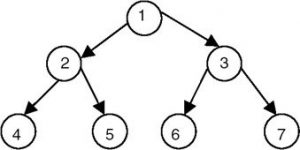# Right sibling of each node in a tree given as array of edges

• Difficulty Level : Easy
• Last Updated : 12 Sep, 2022

Given a tree, with N nodes and E edges (every edge is denoted by two integers, X, Y stating that X is the parent of Y), the task is to print all the nodes with their right siblings in separate lines.
If there is no right sibling for a particular node then print -1 instead.

Examples:In the image shown above, nodes 3, 5, 6, 7 are the right siblings of nodes 2, 4, 5, 6 respectively and the nodes 1, 3 and 7 have no right siblings.

Input: N = 7, E = 6
edges[][] = {{1, 2}, {1, 3}, {2, 4}, {2, 5}, {3, 6}, {3, 7}}
Output: 1 -1
2 3
3 -1
4 5
5 6
6 7
7 -1

Input: N = 5, E = 4
edges[][] = {{7, 8}, {7, 10}, {7, 15}, {10, 3}}
Output: 7 -1
8 10
10 15
15 -1
3 -1

Approach: The main idea is to use a Breadth First Traversal.

• Initially, the root node and ‘-1’ value will be pushed into the queue. After every node from a particular level in the tree has been pushed to the queue, ‘-1’ has to be pushed to make sure that the last node in the level has no right sibling.
• After popping each node from the queue, the node at the front of the queue will always be the right sibling of the popped node.
• If the popped node is valued ‘-1’, it means the current level has been visited and if the queue is not empty it means that previous nodes of this level have at least one child which hasn’t been visited.
• Repeat the above steps while the queue is non-empty.

Below is the implementation of the above approach:

## C++

 `// C++ program to print right siblings``// of all the nodes in a tree``#include ``using` `namespace` `std;` `void` `PrintSiblings(``int` `root, ``int` `N, ``int` `E, vector<``int``> adj[])``{``    ``// boolean array to mark the visited nodes``    ``vector<``bool``> vis(N+1, ``false``);` `    ``// queue data structure to implement bfs``    ``queue<``int``> q;``    ``q.push(root);``    ``q.push(-1);``    ``vis[root] = 1;``    ``while` `(!q.empty()) {``        ``int` `node = q.front();``        ``q.pop();``        ``if` `(node == -1) {` `            ``// if queue is empty then``            ``// the popped node is the last node``            ``// no need to push -1.``            ``if` `(!q.empty())``                ``q.push(-1);``            ``continue``;``        ``}` `        ``// node and its right sibling``        ``cout << node << ``" "` `<< q.front() << ``"\n"``;``        ``for` `(``auto` `s : adj[node]) {``            ``if` `(!vis[s]) {``                ``vis[s] = 1;``                ``q.push(s);``            ``}``        ``}``    ``}``}` `// Driver code``int` `main()``{``    ``// nodes and edges``    ``int` `N = 7, E = 6;``    ``vector<``int``> adj[N+1];` `    ``// The tree is represented in the form of``    ``// an adjacency list as there can be``    ``// multiple children of a node``    ``adj.push_back(2);``    ``adj.push_back(3);``    ``adj.push_back(4);``    ``adj.push_back(5);``    ``adj.push_back(6);``    ``adj.push_back(7);``    ``int` `root = 1;``    ``PrintSiblings(root, N, E, adj);``    ``return` `0;``}`

## Java

 `// Java program to print right siblings``// of all the nodes in a tree``import` `java.util.*;` `class` `GFG``{``static` `void` `PrintSiblings(``int` `root, ``int` `N,``                          ``int` `E, Vector adj[])``{``    ``// boolean array to mark the visited nodes``    ``boolean` `[]vis = ``new` `boolean``[N + ``1``];` `    ``// queue data structure to implement bfs``    ``Queue q = ``new` `LinkedList<>();``    ``q.add(root);``    ``q.add(-``1``);``    ``vis[root] = ``true``;``    ``while` `(!q.isEmpty())``    ``{``        ``int` `node = q.peek();``        ``q.remove();``        ``if` `(node == -``1``)``        ``{` `            ``// if queue is empty then``            ``// the popped node is the last node``            ``// no need to push -1.``            ``if` `(!q.isEmpty())``                ``q.add(-``1``);``            ``continue``;``        ``}` `        ``// node and its right sibling``        ``System.out.print(node + ``" "` `+``                           ``q.peek() + ``"\n"``);``        ``for` `(Integer s : adj[node])``        ``{``            ``if` `(!vis[s])``            ``{``                ``vis[s] = ``true``;``                ``q.add(s);``            ``}``        ``}``    ``}``}` `// Driver code``public` `static` `void` `main(String[] args)``{``    ``// nodes and edges``    ``int` `N = ``7``, E = ``6``;``    ``Vector []adj = ``new` `Vector[N + ``1``];``    ``for``(``int` `i = ``0``; i < N + ``1``; i++)``        ``adj[i] = ``new` `Vector();``        ` `    ``// The tree is represented in the form of``    ``// an adjacency list as there can be``    ``// multiple children of a node``    ``adj[``1``].add(``2``);``    ``adj[``1``].add(``3``);``    ``adj[``2``].add(``4``);``    ``adj[``2``].add(``5``);``    ``adj[``3``].add(``6``);``    ``adj[``3``].add(``7``);``    ``int` `root = ``1``;``    ``PrintSiblings(root, N, E, adj);``}``}` `// This code is contributed by Rajput-Ji`

## Python3

 `# Python3 program to print right``# siblings of all the nodes in``# a tree``def` `PrintSiblings(root, N, E, adj):` `    ``# Boolean array to mark the``    ``# visited nodes``    ``vis ``=` `[``False` `for` `i ``in`  `range``(N ``+` `1``)]``    ` `    ``# queue data structure to``    ``# implement bfs``    ``q ``=` `[]``    ``q.append(root)``    ``q.append(``-``1``)``    ``vis[root] ``=` `1``    ` `    ``while` `(``len``(q) !``=` `0``):``        ``node ``=` `q[``0``]``        ``q.pop(``0``)``        ` `        ``if` `(node ``=``=` `-``1``):`` ` `            ``# If queue is empty then the``              ``# popped node is the last node``            ``# no need to append -1.``            ``if` `(``len``(q) !``=` `0``):``                ``q.append(``-``1``)``            ` `            ``continue``            ` `        ``# Node and its right sibling``        ``print``(``str``(node) ``+` `" "` `+` `str``(q[``0``]))``        ` `        ``for` `s ``in` `adj[node]:``            ``if` `(``not` `vis[s]):``                ``vis[s] ``=` `True``                ``q.append(s)``            ` `# Driver code``if` `__name__``=``=``'__main__'``:` `    ``# Nodes and edges``    ``N ``=` `7``    ``E ``=` `6``    ``adj ``=` `[[] ``for` `i ``in` `range``(N ``+` `1``)]`` ` `    ``# The tree is represented in the``      ``# form of an adjacency list as``    ``# there can be multiple children``    ``# of a node``    ``adj[``1``].append(``2``)``    ``adj[``1``].append(``3``)``    ``adj[``2``].append(``4``)``    ``adj[``2``].append(``5``)``    ``adj[``3``].append(``6``)``    ``adj[``3``].append(``7``)``    ``root ``=` `1``    ` `    ``PrintSiblings(root, N, E, adj)``    ` `# This code is contributed by rutvik_56`

## C#

 `// C# program to print right siblings``// of all the nodes in a tree``using` `System;``using` `System.Collections.Generic;` `class` `GFG``{``static` `void` `PrintSiblings(``int` `root, ``int` `N,``                          ``int` `E, List<``int``> []adj)``{``    ``// bool array to mark the visited nodes``    ``bool` `[]vis = ``new` `bool``[N + 1];` `    ``// queue data structure to implement bfs``    ``Queue<``int``> q = ``new` `Queue<``int``>();``    ``q.Enqueue(root);``    ``q.Enqueue(-1);``    ``vis[root] = ``true``;``    ``while` `(q.Count != 0)``    ``{``        ``int` `node = q.Peek();``        ``q.Dequeue();``        ``if` `(node == -1)``        ``{` `            ``// if queue is empty then``            ``// the popped node is the last node``            ``// no need to push -1.``            ``if` `(q.Count != 0)``                ``q.Enqueue(-1);``            ``continue``;``        ``}` `        ``// node and its right sibling``        ``Console.Write(node + ``" "` `+``                      ``q.Peek() + ``"\n"``);``        ``foreach` `(``int` `s ``in` `adj[node])``        ``{``            ``if` `(!vis[s])``            ``{``                ``vis[s] = ``true``;``                ``q.Enqueue(s);``            ``}``        ``}``    ``}``}` `// Driver code``public` `static` `void` `Main(String[] args)``{``    ``// nodes and edges``    ``int` `N = 7, E = 6;``    ``List<``int``> []adj = ``new` `List<``int``>[N + 1];``    ``for``(``int` `i = 0; i < N + 1; i++)``        ``adj[i] = ``new` `List<``int``>();``        ` `    ``// The tree is represented in the form of``    ``// an adjacency list as there can be``    ``// multiple children of a node``    ``adj.Add(2);``    ``adj.Add(3);``    ``adj.Add(4);``    ``adj.Add(5);``    ``adj.Add(6);``    ``adj.Add(7);``    ``int` `root = 1;``    ``PrintSiblings(root, N, E, adj);``}``}` `// This code is contributed by Rajput-Ji`

## Javascript

 ``

Output

```1 -1
2 3
3 -1
4 5
5 6
6 7
7 -1
```

My Personal Notes arrow_drop_up